treeTS

## Introduction¶

In two earlier posts ( and ), we had two examples of how to build well performing time-series models with relatively lightweight approaches. This time, I'll demonstrate an idea with tree based estimators, again under the premise of keeping the model fairly simple.

In :
import pandas as pd
import numpy as np
import matplotlib.pyplot as plt

from sklearn.tree import DecisionTreeRegressor, export_graphviz
import graphviz


## The dataset¶

To ease comparison with the former approaches, I stuck with the airline passengers dataset from before. Since tree based estimators can only work with stationary data, we need to remove and form of non-stationarity. This is the same problem as with the Naive-Bayes approach from , therefore the preprocessing is the same as for that model.

In :
data = pd.read_csv("passengers.csv", header=0)

In :
data.head()

Out:
Month International airline passengers: monthly totals in thousands. Jan 49 ? Dec 60
0 1949-01 112
1 1949-02 118
2 1949-03 132
3 1949-04 129
4 1949-05 121
In :
data.set_index("Month",inplace=True)

In :
plt.figure(figsize=(12,6))
plt.plot(data.values)

Out:
[<matplotlib.lines.Line2D at 0x1a1d1d15c0>]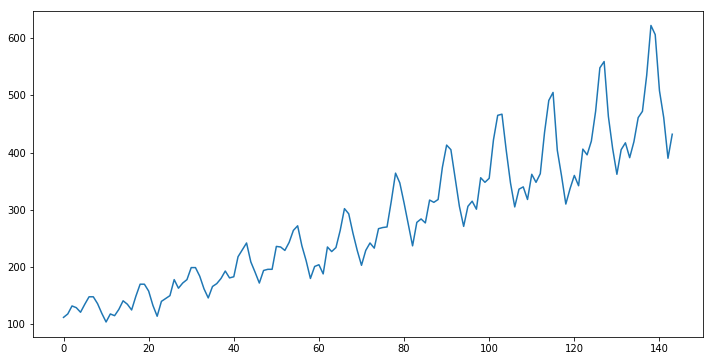In :
train_size = len(data) - 36
test_size = len(data) - train_size
train, test = data.iloc[:train_size], data.iloc[train_size:]

train_diffed = train.diff().dropna().values
test = test.values

t_train = np.arange(len(train_diffed)).reshape(-1,1)
t_test = np.arange(len(train_diffed),test_size+len(train_diffed)).reshape(-1,1)

In :
plt.figure(figsize=(12,6))
plt.plot(train_diffed)

Out:
[<matplotlib.lines.Line2D at 0x1a1d3df0f0>]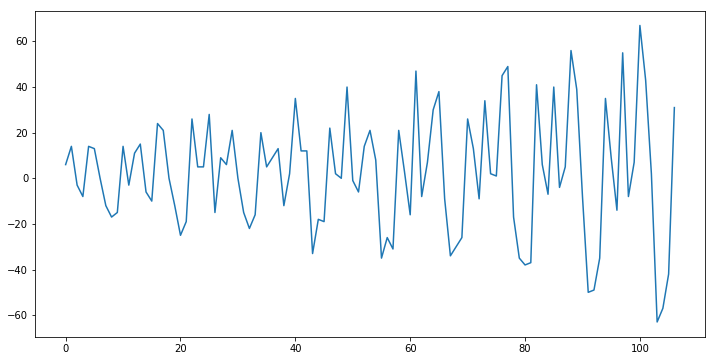In :
trend_removed = train_diffed.reshape(-1) / ((t_train+1)**(1/2)).reshape(-1)

plt.figure(figsize = (12,6))
plt.plot(trend_removed)

Out:
[<matplotlib.lines.Line2D at 0x1a1d53f4a8>]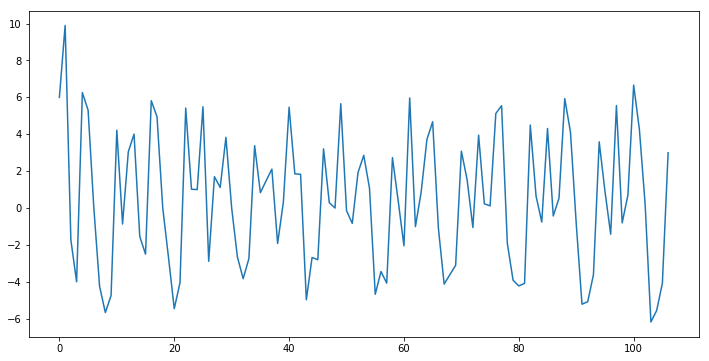In :
train_full = trend_removed[5:]
t_train = np.arange(len(train_full)).reshape(-1,1)
t_test = np.arange(len(train_full),test_size+len(train_full)).reshape(-1,1)


## The model¶

Now comes the fun part. As an interesting twist, we will stay completely in the time-domain for the depending variables and won't employ any sort of autoregressive approach - i.e. we will not regress the present realization on past realizations like

$$X_t=f(X_{t-1},X_{t-2},...,X_1)$$

but rather go with

$$X_t=f(t)$$

The challenge when trying to use a tree model to regress on the time-index, is obviously the continuous increase of $t$ once we leave the training data. Imagine building a Decision Tree with data from periods $[1,50]$ and want to forecast periods $[51,75]$. Per inductive bias of the tree algorithm, predictions for times outside of the training period will be flat:

In :
tree_model = DecisionTreeRegressor(max_depth=3, random_state=123)
tree_model.fit(t_train, train_full)

pred = tree_model.predict(t_test)
pred_mean = np.full(36,np.mean(train_full))

plt.figure(figsize=(12,6))
plt.plot(np.concatenate([train_full, pred]), label="Training data")
plt.plot(np.arange(len(train_full),36+len(train_full)), pred, label="Out of sample forecast")
plt.plot(np.full(int(len(train_full)+36),np.mean(train_full)), label="Unconditional Mean")
plt.legend()

Out:
<matplotlib.legend.Legend at 0x1a1d5a5c50>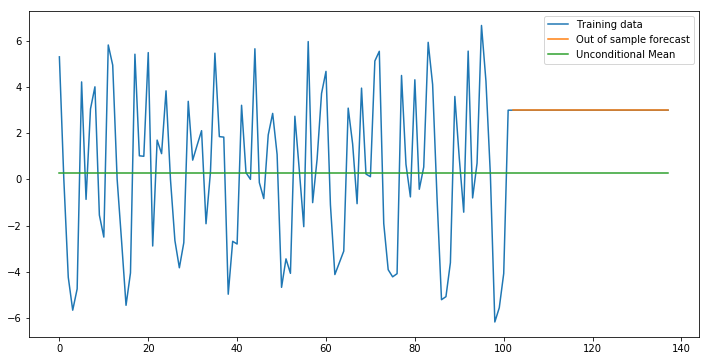In fact, each future time-index will fall into the exact same leaf where

$$t_{future}>path\,with\,largest\,time\,index\,among\,all\,binary\,splits$$

While we could assume that the flat line is the best possible prediction, we likely miss out on the obviously recurring patterns in the time-series. Also, the height of the line seems to be a much worse predictor than the unconditional mean of the time-series - not a good model so far.

What we want is to somehow express the periodic patterns in our time variable and use those in our tree model. A first solution would be to create new features by squashing the time-index through (co-)sine functions with different frequencies $p$:

$$g_{sin}(t)=sin(p\cdot t)$$
$$g_{cos}(t)=cos(p\cdot t)$$

This might indeed make sense and be a valid solution. However there is an even easier way that avoids having to find the right frequencies and - as a bonus - allows to make the resulting Decision Tree interpretable (as long as its size is small enough to be human readable).

The simple trick here is to create new features by using the modulo operator on $t$:

$$g_i^*(t)=t\,mod\,i,\quad i\in\mathbb{Z}^+$$

By doing so, we project time onto an integer circle that gets traversed every $i$ periods. We then have

$$g_i^*(t)\in\{0,...,i-1\}\quad\forall t$$

regardless of whether we are in the training or forecasting period. We can then create multiple features $g_i^*(t)$ features by varying $i$ over some range. Let's implement the proposed procedure:

In :
mod_train = np.concatenate([t_train%t for t in range(1,37)],1)
mod_test = np.concatenate([t_test%t for t in range(1,37)],1)

In :
np.random.seed(123)
tree_model = DecisionTreeRegressor(max_depth=3, random_state=123)
tree_model.fit(mod_train, train_full)

Out:
DecisionTreeRegressor(criterion='mse', max_depth=3, max_features=None,
max_leaf_nodes=None, min_impurity_decrease=0.0,
min_impurity_split=None, min_samples_leaf=1,
min_samples_split=2, min_weight_fraction_leaf=0.0,
presort=False, random_state=123, splitter='best')
In :
pred_tree = tree_model.predict(mod_test)

In :
plt.figure(figsize=(12,6))
plt.plot(np.concatenate([train_full, pred_tree]), label="Training data")
plt.plot(np.arange(len(train_full),36+len(train_full)), pred_tree, label="Out of sample forecast")
plt.plot(pred_mean, label="Unconditional Mean")
plt.legend()

Out:
<matplotlib.legend.Legend at 0x1a1d728ba8>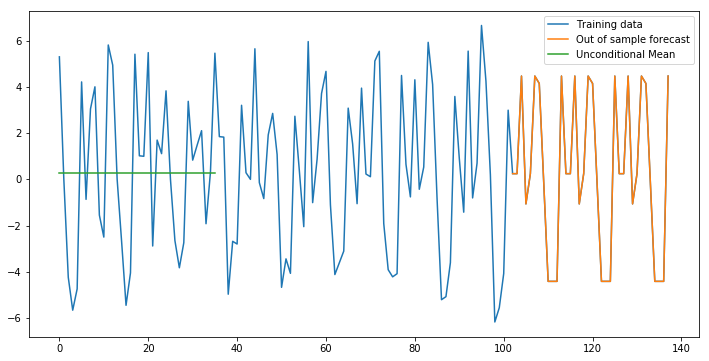The forecast looks reasonable - the obvious patterns from the transformed training data seem to be recognized by our model. Now we can compare the evaluate on our actual test set (of course, we need to invert the initial transformation of our dataset first).

In :
pred = (np.cumsum(pred_tree)*((t_test+1)**(1/2)).reshape(-1) + train.iloc[-1,0])
pred_mean = (np.cumsum(pred_mean)[:36]*((t_test+1)**(1/2)).reshape(-1) + train.iloc[-1,0])

In :
plt.figure(figsize=(12,6))
plt.plot(test, label = "Out of sample test data")
plt.plot(pred, label = "Forecast")
plt.plot(pred_mean, label = "Unconditional mean")
plt.legend()

Out:
<matplotlib.legend.Legend at 0x1a1d894a20>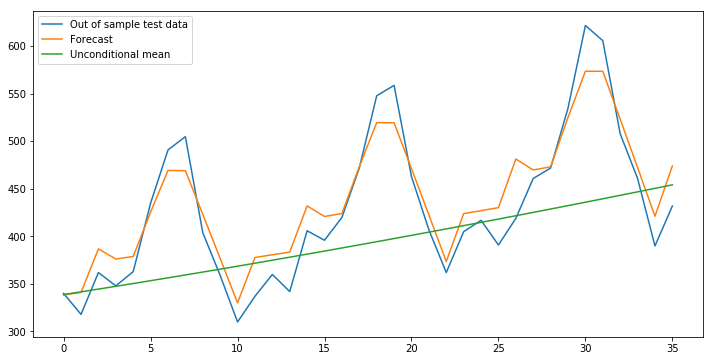In :
np.sqrt(np.mean((pred - test.reshape(-1))**2))

Out:
26.69749158609182
In :
np.sqrt(np.mean((pred_mean - test.reshape(-1))**2))

Out:
75.27880388952589

The result on the test set are looking fine - our model clearly outperforms the naive forecast and is close to the former Naive Bayes model. Now let's output the exact model that the Decision Tree has learnt:

In :
graphviz.Source(export_graphviz(tree_model, out_file = None, feature_names = ["Period=%s" %(i) for i in range(1,37)]))

Out:

We can see that the model learnt the rather obvious yearly pattern (Period=12) another reasonable quarterly (Period=3) and a pattern that repeats itself every four months (Period=4). Interestingly, the model also learnt two patterns that aren't as obvious as the other three, namely a Period=13 and a Period=25 pattern. Although these two patterns don't contribute as much to the reduction of MSE in each node, it might be interesting to use this knowledge for further modeling.

## Conclusion¶

In this rather short post, we looked at a reasonable way to use a Decision Tree for time-series forecasting. The proposed approach can be made quite sparse in terms of model parameters - in the simplest case we could go with a Decision Tree stump that splits the input space only once. This can be quite advantageous for time-series problems where the amount of available training data is small as well in order to avoid overfitting. On the other hand is of course the easy interpretability of tree models that allows us to easily explain our forecasts to potential stakeholders. Obviously, we could also add an autoregressive component or external regressors here to make our model more powerful. To enhance our predictive power while keeping the model interpretable, we could switch over to the RuleFit algorithm which I explained here and also applied to a non time-series problem here.